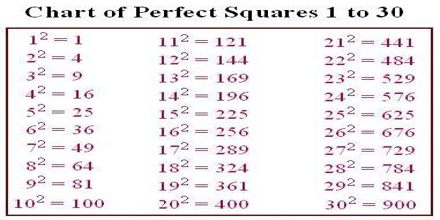Mathematic

# Squares and Square RootsPrinciple objective of this lecture is to present on Squares and Square Roots. A square is a regular quadrilateral, which means that it has four equal sides and four equal angles (90-degree angles, or right angles). Square Roots are one of the two equal factors of a number. A square root of 144 is 12 since 12 squared = 144. here also describe on Types of Triangles: Acute: triangles with angles less than 90º; Obtuse: triangles with one angle greater than 90º but less than 180º and Right: triangles with one angle equaling 90º.